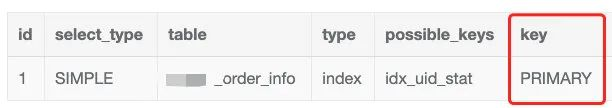# 这个 MySQL bug 99% 的人会踩坑!

Hunter37

0收藏

``select * from order_info where uid = 5837661 order by id asc limit 1``

``````CREATE TABLE `order_info` (
`id` bigint(20) unsigned NOT NULL AUTO_INCREMENT,
`uid` int(11) unsigned,
`order_status` tinyint(3) DEFAULT NULL,
... 省略其它字段和索引
PRIMARY KEY (`id`),
KEY `idx_uid_stat` (`uid`,`order_status`),
) ENGINE=InnoDB DEFAULT CHARSET=utf8``````

``explain select * from order_info where uid = 5837661 order by id asc limit 1````````SET optimizer_trace="enabled=on";        // 打开 optimizer_trace
SELECT * FROM order_info where uid = 5837661 order by id asc limit 1
SELECT * FROM information_schema.OPTIMIZER_TRACE;    // 查看执行计划表
SET optimizer_trace="enabled=off"; // 关闭 optimizer_trace``````

MySQL 优化器首先会计算出全表扫描的成本，然后选出该 SQL 可能涉及到的所有索引并且计算索引的成本，然后选出所有成本最小的那个来执行，来看下 optimizer trace 给出的关键信息

``````{
"rows_estimation": [
{
"table": "`rebate_order_info`",
"range_analysis": {
"table_scan": {
"rows": 21155996,
"cost": 4.45e6    // 全表扫描成本
}
},
...
"analyzing_range_alternatives": {
"range_scan_alternatives": [
{
"index": "idx_uid_stat",
"ranges": [
"5837661 <= uid <= 5837661"
],
"index_dives_for_eq_ranges": true,
"rowid_ordered": false,
"using_mrr": false,
"index_only": false,
"rows": 255918,
"cost": 307103,            // 使用idx_uid_stat索引的成本
"chosen": true
}
],
"chosen_range_access_summary": {    // 经过上面的各个成本比较后选择的最终结果
"range_access_plan": {
"type": "range_scan",
"index": "idx_uid_stat",  // 可以看到最终选择了idx_uid_stat这个索引来执行
"rows": 255918,
"ranges": [
"58376617 <= uid <= 58376617"
]
},
"rows_for_plan": 255918,
"cost_for_plan": 307103,
"chosen": true
}
}
...``````

``````{
"reconsidering_access_paths_for_index_ordering": {
"clause": "ORDER BY",
"index_order_summary": {
"table": "`rebate_order_info`",
"index_provides_order": true,
"order_direction": "asc",
"index": "PRIMARY",    // 可以看到选择了主键索引
"plan_changed": true,
"access_type": "index_scan"
}
}
}``````

The short explanation is that the optimizer thinks — or should I say hopes — that scanning the whole table (which is already sorted by the id field) will find the limited rows quick enough, and that this will avoid a sort operation. So by trying to avoid a sort, the optimizer ends-up losing time scanning the table.

1.使用 force index 来强制使用指定的索引，如下：

``select * from order_info force index(idx_uid_stat) where uid = 5837661 order by id asc limit 1``

2.使用 order by (id+0) 方案，如下

``select * from order_info where uid = 5837661 order by (id+0) asc limit 1``

• mysql 优化器 bug http://4zsw5.cn/L1zEi